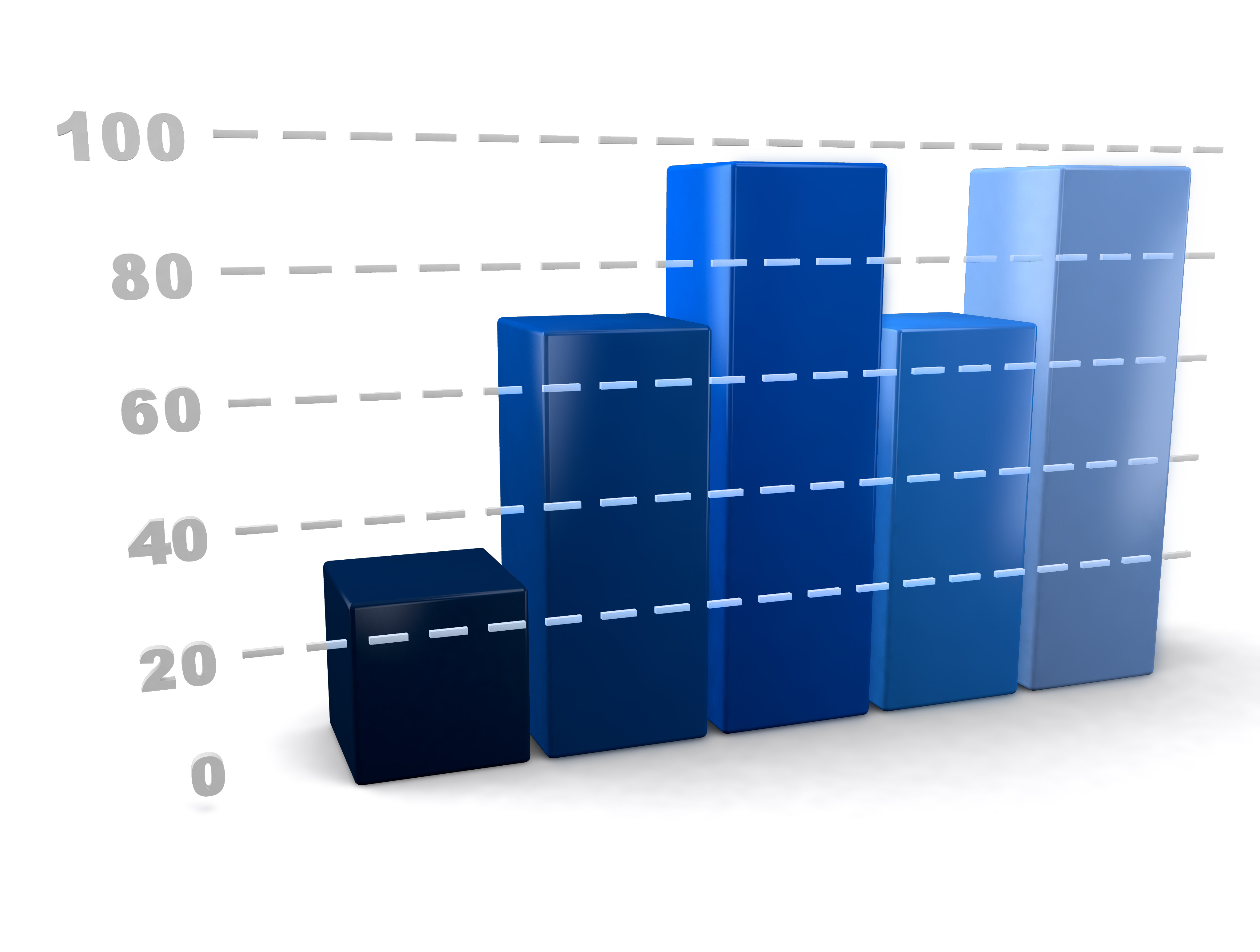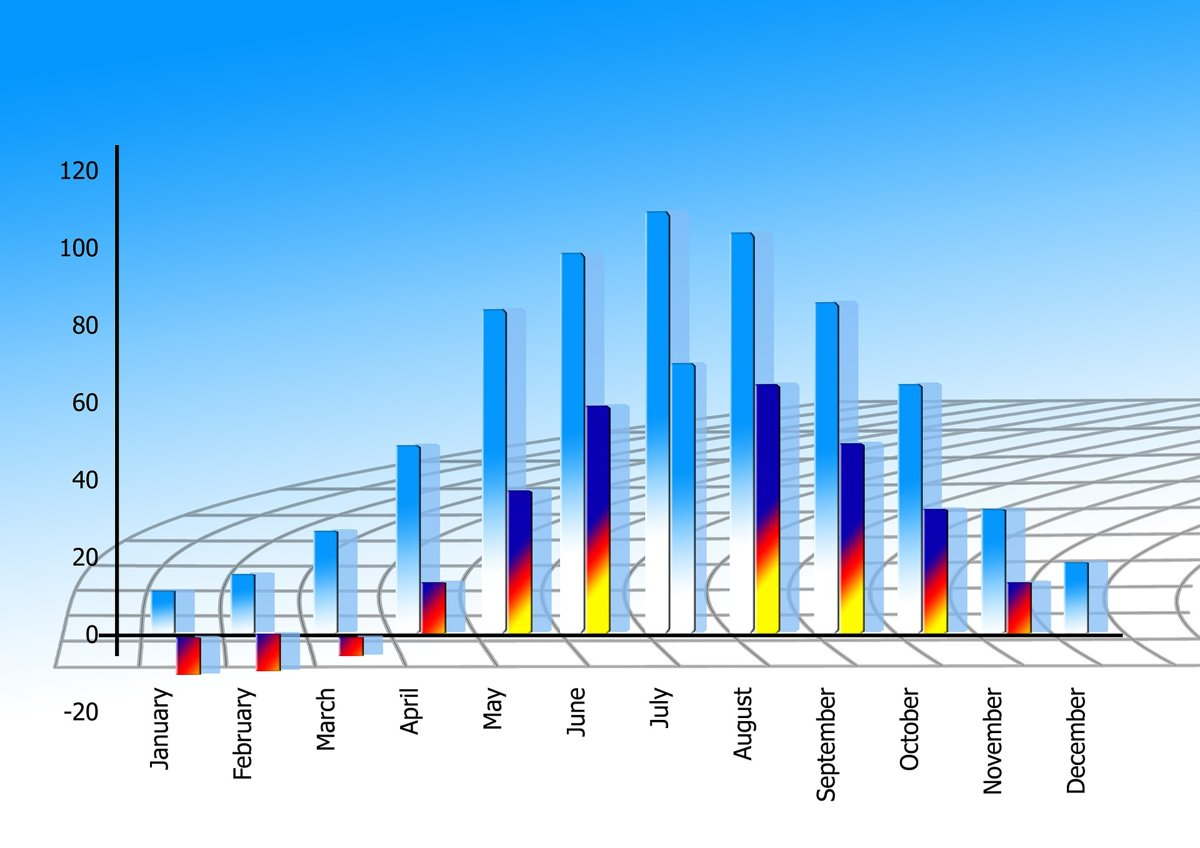# Baud Rate in Arduino Serial Communication

Yousef Zahid May 14, 2021

Arduino coding is quite simple if one understands the basic concept and has the knack for logical implementation. This concept discussed in this article will be related to the Baud rate. Most novice Arduino coders use a specific Baud rate value for all of their programs and functions but are unaware of why a value of `9600` is used rather than another number. What makes this value special, and what is the actual use of the Baud rate?

Baud rate is simply the rate of signal or symbol change per second. It quite hard to understand just from the definition. A more visual interpretation would be better.Let us consider the Bar chart in figure 1. Assume every block has a period of 1 second. As the period is about to end, there is an abrupt change in the value of the bar chart. For the first bar, it suddenly increases in value. This change per second is known as the baud rate, and the baud rate in this particular case is 1. The signal changed once per second.For this new graph, consider the month of January in which the signal changes two times till February: this represents a baud rate of two. It also means that a port can transmit a maximum of about 2 bits per second. If the baud rate increase beyond `76800` units, then the wire length needs to be reduced for optimum performance.

There is a subtle difference between baud rate and bit rate. Bit rate is the transmission rate of bit per second, while baud rate is the signal or symbol changes per second. The bit rate can be greater than the baud rate, but not vice versa. Baud rates are associated with electronics, especially DIY projects involving Arduino development board.

To use it as a global function in programming via C++, we need to assign an intended value with `#define BAUDRATE` for use later on. If you want to send and receive data at a faster rate, then you must increase the value accordingly; however, for a microcontroller, the typical value is `9600`.

Now the selection criteria depend upon a lot of factors. First of all, if you want to increase the bit rate, you must increase the baud rate.

The oversampling criteria must adhere to match the baud rates; even though one can change the oversampling rate from `16X` to `8X` or vice versa for which tight tolerance clocks are required, an error might exist. Reducing the rate will increase the error, and the start bit edge might not match with the received bit edge.